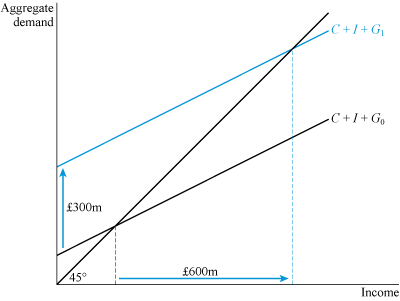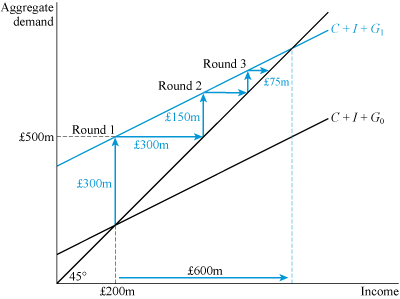Economics and the 2008 crisis: a Keynesian view

Start this free course now. Just create an account and sign in. Enrol and complete the course for a free statement of participation or digital badge if available.

6.4 Government expenditure (4)

The aggregate demand model can also be used to illustrate what might happen under an increase in government spending, as shown in Figure 15. Let government spending increase by £300 million – money that could be spent along the lines of the 2009 Obama Recovery Act or the 1929 Lloyd George three-point plan. This is shown by an upward shift of the intercept of the aggregate demand schedule by £300 million due to an increase in government spending from G0 to G1. It is referred to in modern economics as a fiscal stimulus. Using one of its key fiscal powers – the ability to carry out expenditure – the government is able to boost aggregate demand.Figure 15 Modelling a fiscal stimulus

Activity 10

By how much does income increase in Figure 15 under a £300 million fiscal stimulus?

Figure 15 shows, for this particular example, that the increase in income is £600 million – which is double the fiscal stimulus. In this example an extra £1 million of income is created for each £1 million of fiscal stimulus – an extra £1 million of output that could lead to double the number of jobs initially created directly and indirectly by the stimulus.

This ‘double your money’ insight can now be explained by breaking down the effect of the stimulus into a series of rounds, as shown in Table 1. In Round 1, the change in income is equal to the fiscal stimulus of £300 million. Round 2 then depends on how much of this income is spent.

Table 1 Direct and indirect impacts of a fiscal stimulus

RoundChange in income (£ m)Cumulative change in income (£ m)
1300.00300.00
2150.00450.00
375.00525.00
437.50562.50
518.75581.25
69.38590.63
74.69595.32
82.35597.67
91.18598.85
100.59599.44

It is modestly assumed in Figure 15 that the marginal propensity to consume – the slope (b) of the consumption function – is equal to 1/2. This means that in Round 2, half of the initial increase in income from the fiscal stimulus will be spent on consumption, as shown by the £150 million change in income. By Round 2 it therefore follows that there is a cumulative increase in income of (i) the initial direct increase in income of £300 million, and (ii) the additional indirect impact of £150 million. These two items add up to a cumulative income of £450 million in Round 2.

This process continues in Round 3, with half of the previous round’s change in income (£150 million) spent as consumption: a new change in income of £75 million. In each round, half of the previous round’s change in income is spent; these rounds continue indefinitely. By Round 10, however, the change in income has fallen to just over half a million pounds, and the cumulative change approaches its final value of £600 million – the doubling of income that is illustrated in Figure 15. Even by Round 3, 87.5% of the eventual cumulative change in income has occurred, so although in theory the expansionary effect may take many rounds and a great deal of time to work itself out, the main impact is felt quite quickly.

Figure 16 shows the first three rounds. In Round 1, the injection of government demand leads to an upward shift of the aggregate demand schedule by £300 million. Initially, this planned aggregate demand exceeds current output. At the outset, in Round 1, only £200 million worth of output (income) is being produced, yet now the total aggregate demand is £500 million. If the key assumption is now made that there is sufficient spare capacity – idle factories or machinery, and a pool of unemployed workers – then in response to the additional demand, output can be increased by £300 million, as depicted by the first horizontal arrow.Figure 16 Direct and indirect impacts of a fiscal stimulus

In Round 2, the new stimulus of £150 million, as shown in Table 1, comes into play. Once again aggregate demand exceeds current output, this time by £150 million, and a new horizontal line indicates the increase in output to match this demand. Similarly, in Round 3 the impact of the £75 million boost in aggregate demand is matched by a £75 million boost in output. These rounds eventually peter out, as in Table 1, when both aggregate demand and aggregate output have increased by £600 million.

The process, illustrated in Table 1 and in Figure 16 is referred to by Keynes as the multiplier process, since the impact of the fiscal stimulus is multiplied throughout the economy. The multiplier captures the cumulative effect of a change in spending on income. The change represented here is a change in government spending, but the model could also be used to show the effect of a change in another exogenous factor such as investment.

Let Δ denote the amount by which a variable might change. The multiplier can be expressed in mathematical terms as the ratio of the eventual change in income (ΔY ) to the initial change in spending that caused it (ΔG ):

Activity 11

Calculate the size of the multiplier when a £300 million fiscal stimulus increases income by £900 million.

In this example, ΔY = 900 and ΔG = 300; hence the multiplier is equal to 900/300 = 3. Since the multiplier is 3, the fiscal stimulus increases income by a threefold amount.

The size of the multiplier depends on the marginal propensity to consume, as explored in the box below. It has a simple formula:

where b is the marginal propensity to consume. In the example in Table 1, the propensity to consume is equal to 1/2. Hence

The eventual doubling of income in the multiplier process is captured in total by this formula for the multiplier.

Since the concept of the multiplier emerged in the 1930s, economists have used statistical methods to estimate its empirical size. There is, however, considerable uncertainty as to the multiplier’s precise value. At the top end of estimates of the size of the multiplier, Eggertsson (2006) finds the multiplier to be as high as 3.8. In a more recent survey, Ramey (2011) pronounces that the ‘range of plausible estimates for the multiplier … is probably 0.8 to 1.5’. Due to the uncertainty involved, however: ‘Reasonable people could argue that the multiplier is 0.5 or 2.0’. A multiplier of 0.5 would mean, for example, that £1 billion of spending leads to only half that increase in income; whereas a multiplier of 2.0, as you have seen, would mean a doubling of income.

One problem is that estimates tend to be based on observations made during periods of economic growth, yet multipliers are most relevant in times of contraction. Larry Summers, a leading US economist who has advised Presidents Clinton and Obama, has been very cautious about the impact of fiscal stimuli but argues that the specific circumstances of the economic crisis of 2008 would point to a multiplier nearer to the top end of Ramey’s range of estimates (DeLong and Summers, 2012).

Deriving the formula for the multiplier

To derive the formula for the multiplier, consider two equations.

First, consider an income equation showing points of equilibrium between income and aggregate demand (points where the aggregate demand schedule intersects the 45-degree line):

Second, consider the consumption function, showing the relationship between planned consumption and income:

Substituting the consumption function into the income equation gives a new expression:

Now notice that on the right-hand side there is an expression that relates consumption to income. I can now subtract this term from both sides of the expression:

Collecting 1 and b in a bracket:

Dividing both sides of this equation by (1 − b), this term cancels out on the left-hand side, to leave:

This gives a multiplier relationship between income and exogenous spending

The expression is the multiplier below: